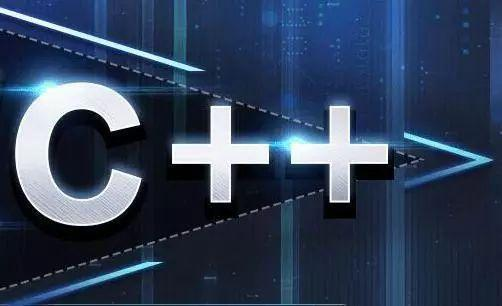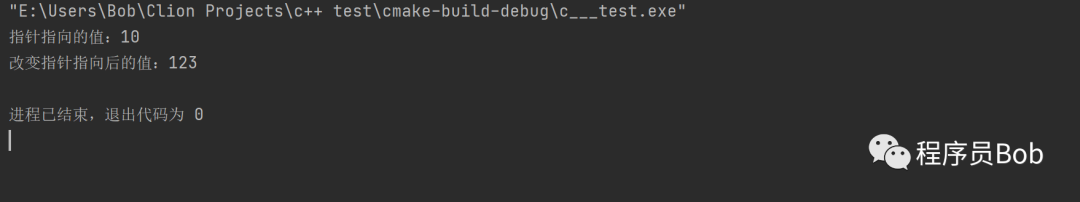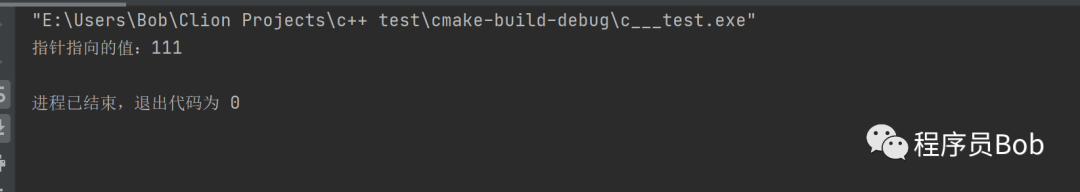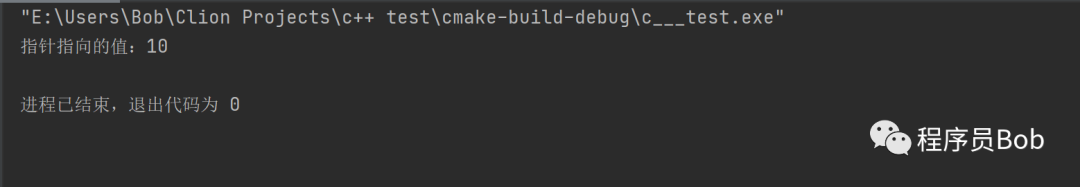# const 与指针交集的那些事Bob# 1.const 修饰指针

#include <iostream>using namespace std;int main() {int a,b;    a=10;    b=123;const int *p=&a;//const修饰指针，指针指向的值不可以改，指针的指向可以改// *p=20;错误cout <<"指针指向的值："<< *p <<endl;    p=&b;cout <<"改变指针指向后的值："<< *p <<endl;return 0;}# 2.const 修饰常量

#include <iostream>using namespace std;int main() {int a,b;    a=10;    b=123;//const修饰常量//指针的指向不可以改，指针指向的值可以改int *const p1=&a;    *p1=111;//p2=&b;错误cout <<"指针指向的值："<< *p1 <<endl;return 0;}# 3.const 修饰指针和常量

#include <iostream>using namespace std;int main() {    int a,b;    a=10;    b=123;    //const修饰指针和常量    //指针指向和指向的值都不可以更改    const int *const p2=&a;    //p3=&b;错误    //*p3= 30;错误    cout <<"指针指向的值："<< *p2 <<endl;    return 0;}## 评论